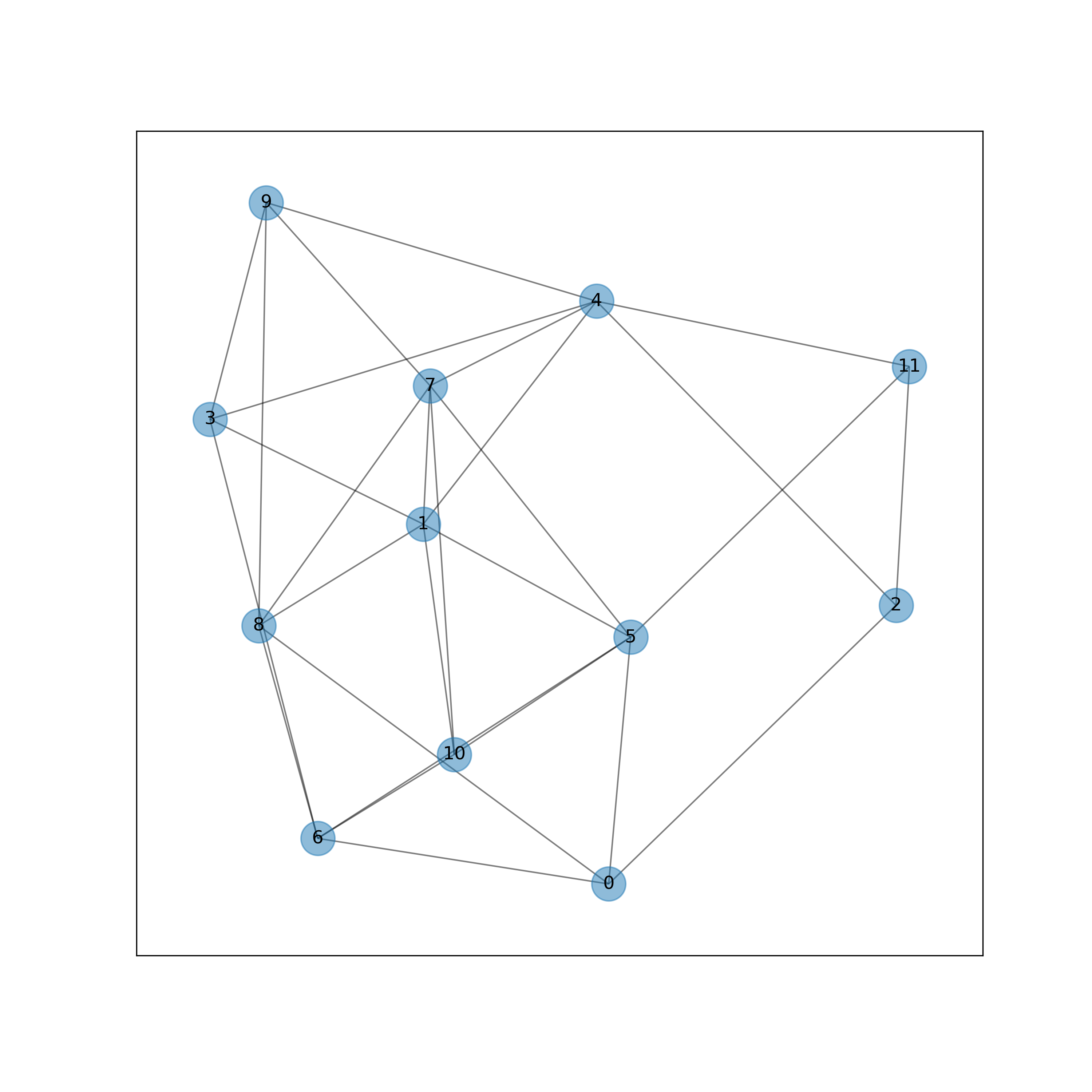Breadth-first search (BFS) is a traversing algorithm for unweighted graphs. This is a foundational algorithm in graph theory from which many other algorithms start.

## Features

Some of the features and constraints that define the use and functionality of a breadth-first search algorithm include the following:

• A relatively simple and efficient algorithm for finding the shortest-path in graphs that do not have edge weights.
• It has a time complexity of O(|V| + |E|), which is the sum of the vertices and edges.
• In addition to finding the shortest path between two nodes, this algorithm can be used to map or traverse a graph to find all connections or if a given path exists between any pair of nodes.

## Implementation

The example below walks through a basic implementation of BFS that will take a starting node, an end node, and a graph as arguments. However, to start, a brief overview of the necessary steps or pseudocode will serve as an outline for the final implementation.

The BFS algorithm is built upon a simple process of elimination. It begins with the selection of a node and the identification of all the neighboring nodes. The algorithm iterates through these basic steps until all nodes have been evaluated (or another objective has been achieved). Through this process, the graph is explored in a layered approach where each node at a given level (or degree) is evaluated before moving on to the next. Implicitly, a search employing this process will yield the shortest path between any two nodes.

As noted in the description above, the BFS process is an iterative one. The implementation commonly revolves around adding and removing nodes as their evaluated from a queue.

The implementation below can be broken down into the following steps:

• A list is initialized with the starting node, and nodes will be added to and removed from this list in a queue-like manner.
• Another list is initialized for tracking nodes that have already been processed.
• A variable is initialized for tracking the path (the sequence of nodes from the source to the final node).
• A variable is initialized to represent the current node being evaluated (the last item in the path).
• A loop is set to iterate over items in the list/queue.
• The process starts in earnest by iterating over all edge-pairs to identify the starting node.
• If the starting node is present in an edge-pair, as the source or destination, then the accompanying (neighboring) node is added to the `neighbors` list.
• Once all the neighbors have been collected, another loop iterates over this list.
• A second variable for updating the path (`curr_path`) is instatiated. This variable is the sum of the existing steps in addition to the current node.
• Each neighbor is tested. If it matches the destination node, the search is complete and `curr_path` is returned.
• If the neighbor is not a match, the path is added to the queue.
• Once all the neighbors have been exhausted, the `curr_node` is added to the tested nodes list. Now, the process starts again from the top with the next path in the queue.
• The loop iterates until the queue is empty or until a path is returned.
• If all the nodes are processed and there is no path, an empty list is returned.

## Example

The example below generates a random graph and solves the shortest path between two nodes. For the sake of succinctness, the example below leverages the Python library, `networkx`, for generating a random graph. This library is available by default within Google Colaboratory and can serve as a convenient environment for reproducing this example.

```# Imports and graph setupimport networkx as nxfrom matplotlib import pyplot as pltplt.rcParams["figure.figsize"] = (10,10)
# Generating a random graphG = nx.random_graphs.fast_gnp_random_graph(12, 0.4, seed=15)
# Displaying the graphdef draw_graph(G):    pos = nx.spring_layout(G)    nx.draw_networkx_nodes(G, pos, node_size=500, alpha=0.5)    nx.draw_networkx_labels(G, pos)    nx.draw_networkx_edges(G, pos, width=1.0, alpha=0.5)
draw_graph(G)
```

This will yield the following output:Finally, the BFS code is as follows:

```# The function implementing the BFS algorithmdef get_shortest_path(graph_data, node_one, node_two):    node_q = [[node_one]]    tested_nodes = []
while node_q:        path = node_q.pop(0)        curr_node = path[-1]
if curr_node not in tested_nodes:            neighbors = []
for pair in list(graph_data.edges): # The edges attribute provides a list of tuples containing all edge pairs                if curr_node in pair:                    neighbors.append(pair) if pair != curr_node else neighbors.append(pair)
for n in neighbors:
curr_path = path + [n]                if n == node_two:                    return curr_path
node_q.append(curr_path)
tested_nodes.append(curr_node)
return []
# Testing the BFS functionget_shortest_path(G,2,10)
```

Which will yield:

```[2, 0, 5, 10]
```